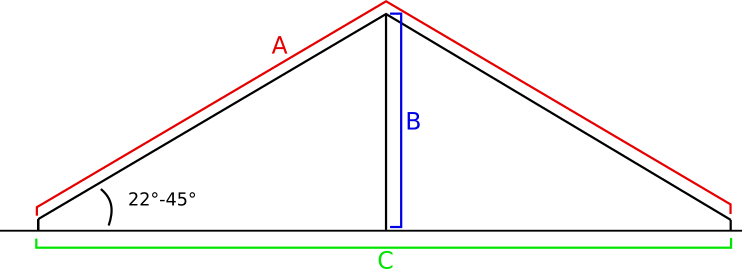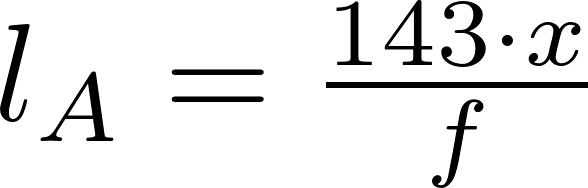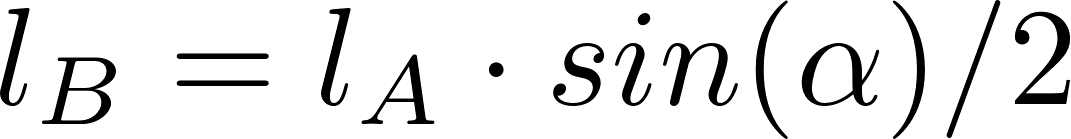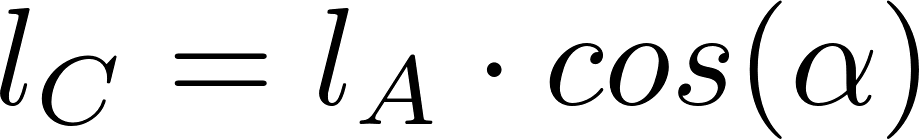# Inverted vee antenna

The inverted vee antenna looks like an upside down V, often at a 120 or 90 degree angle. The antenna has a rod in the center to support the V shape. The inverted vee antenna takes up less space than a standard dipole antenna operating at the same frequency, so it can be a good alternative when less space is available.

### FormulasSchematicl is the symbol for length and is measured in meter (m).
f is the frequency in megahertz (MHz).
x is the length multiplication (e.g. if the total length is 2% shorter, x = 0.98). The values for 22°, 30°, 37° and 45° are 0.98, 0.97, 0.96 and 0.95 respectively.